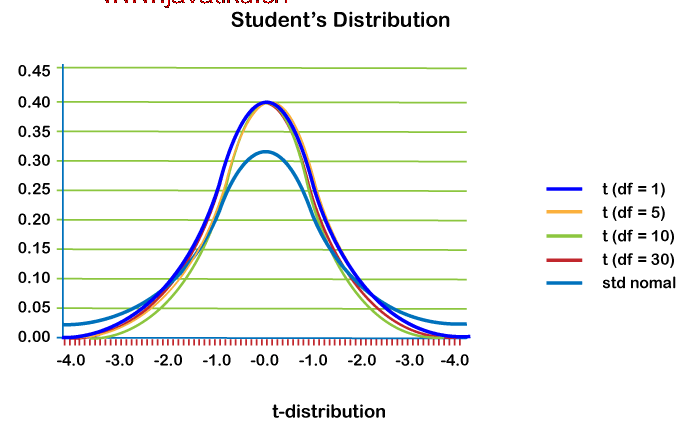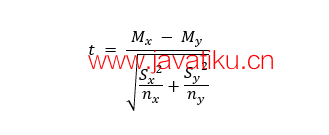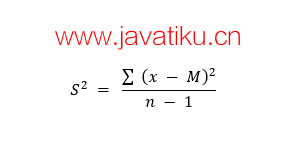# Python教程-Python中的T检验### 简介

T检验是统计学中最常用的程序之一。然而，即使经常使用T检验的许多人也不清楚他们的数据在背景中被处理时会发生什么，使用诸如R和Python等应用程序。

### 理解T检验

T检验是一种比较两个平均值（也称为均值）的测试，它告诉我们它们是否相互不同。T检验也被称为学生T检验，它还告诉我们这些差异有多显著。换句话说，它为我们提供了这些差异是否可能是偶然发生的信息。### 理解T值

T值是两组之间的差异与组内差异之间的比率。如果T值更大，这意味着组之间存在更大的差异。与此同时，较小的T值表示组之间的相似性更大。T值为三（3）表示两组之间相差三倍，以及在各自组内。当我们在进行T检验时得到更大的T值时，结果更有可能是可重复的。

1. 大T值意味着组之间存在差异。
2. 小T值意味着组之间相似。

### T检验的类型有哪些？

T检验有三种重要的类型：

1. 独立样本T检验： 用于比较两组的平均值或均值。
2. 配对样本T检验： 用于比较同一组在不同时间的均值（例如，相隔一年）。
3. 单样本T检验： 用于测试单一组的均值是否与已知均值相同。

## 执行样本T检验

### 确定零假设和备择假设

1. 零假设： 男女身高相同。
2. 备择假设： 男性身高与女性身高不同。

### 确定置信区间和自由度### 计算T统计量M = 均值

n = 每组的分数数x = 个体分数

M = 均值

n = 组内的分数数

### 将关键T值与计算的T统计量进行比较

``````# Importing the required libraries and packages
import numpy as np
from scipy import stats
# Defining two random distributions
# Sample Size
N = 10
# Gaussian distributed data with mean = 2 and var = 1
x = np.random.randn(N) + 2
# Gaussian distributed data with mean = 0 and var = 1
y = np.random.randn(N)
# Calculating the Standard Deviation
# Calculating the variance to get the standard deviation
var_x = x.var(ddof = 1)
var_y = y.var(ddof = 1)
# Standard Deviation
SD = np.sqrt((var_x + var_y) / 2)
print("Standard Deviation =", SD)
# Calculating the T-Statistics
tval = (x.mean() - y.mean()) / (SD * np.sqrt(2 / N))
# Comparing with the critical T-Value
# Degrees of freedom
dof = 2 * N - 2
# p-value after comparison with the T-Statistics
pval = 1 - stats.t.cdf( tval, df = dof)
print("t = " + str(tval))
print("p = " + str(2 * pval))
## Cross Checking using the internal function from SciPy Package
tval2, pval2 = stats.ttest_ind(x, y)
print("t = " + str(tval2))
print("p = " + str(pval2))  ``````

``````Standard Deviation = 0.7642398582227466
t = 4.87688162540348
p = 0.0001212767169695983
t = 4.876881625403479
p = 0.00012127671696957205``````